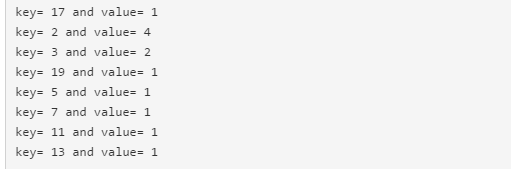# 欧拉计划第5题

## 原题：

2520 is the smallest number that can be divided by each of the numbers from 1 to 10 without any remainder.

What is the smallest positive number that is evenly divisible by all of the numbers from 1 to 20?

## 中文大意：

2520是最小能被1到10整除的数。请问最小能被1到20整除的正整数是多少？

36=2*2*3*3
12=2*2*3

## 代码：

 import java.util.*;

public class MyClass {

public static void main(String args[]){
Map<Integer,Integer> map = new HashMap<Integer,Integer>();//哈希图，保存每个质因数和出现的次数
int num = 0;//保存质因数出现的次数
int n;//当前要被分解的数
//分解11到20
for(int i = 11;i<=20;i++){
n = i;
num = 0;//每次分解将质因数出现次数重置为0
for(int j = 2;j <= n;j++){
//外面的if/else确定能否被整除，能被整除则是质因数
if(n%j == 0){
n = n/j;
num++;
if(map.containsKey(j)==true){
if(num >map.get(j) )//如果原来map中有这个质因数，但这次分解的数产生的质因数出现次数比原来的多，则更新
map.put(j,num);
}
else//map中未存这个质因数，则存入
map.put(j,num);
j--;
}
else
num = 0;
}

}
System.out.println("通过Map.entrySet遍历key和value");
for (Map.Entry<Integer, Integer> entry : map.entrySet()) {
System.out.println("key= " + entry.getKey() + " and value= " + entry.getValue());
}
}
}

## 还是懒：232792560